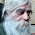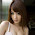# RUDN Mathematicians Confirmed the Possibility of Data Transfer via Gravitational Waves

RUDN mathematicians analyzed the properties of gravitational waves in a generalized affine- metrical space (an algebraic construction operating the notions of a vector and a point) similarly to the properties of electromagnetic waves in Minkowski space-time. It turned out that there is the possibility of transmitting information with the help of nonmetricity waves and transferring it spatially without distortions.

The discovery can help the scientists master new means of data transfer in space, e.g. between space stations. The article of the scientists was published in the Classical and Quantum Gravity journal.

The recently discovered gravitational waves are waves of curvature of space-time, which according to Einstein's general relativity theory is completely determined by the space-time itself. However, currently there are reasons to consider space-time as a more complex structure with additional geometrical characteristics such as torsion and nonmetricity. In this case, geometrically speaking, space-time turns from a Riemannian space envisaged by the General Relativity (GR) into a generalized affine - metrical space. Respective gravitational field equations that generalize Einstein's equations show that torsion and nonmetricity can also spread in the form of waves (in particular plane waves at a great distance from wave sources).

In order to describe gravitational waves RUDN researchers used mathematical abstraction - an affine space, i.e. a usual vector space but without an origin of coordinates. It was proved that in such mathematical representation of gravitational waves there are functions which remain invariable in process of distribution of a wave. It is possible to set arbitrary function so that it encoded any information - in approximately same way electromagnetic waves transfer a radio signal. It means that if to find a way to set these constructions in a wave source, they will be reach any point of space without changes. Thus, gravitational waves could be used for data transfer.

The study consisted of three stages. On the first one RUDN mathematicians calculated the Lie derivative - a function that binds the properties of bodies in two different spaces: an affine space and a Minkowski space. It allowed them to pass from the description of waves in the real space to their mathematical interpretation. At the second stage the researchers determined five arbitrary functions of time, i.e. the constructions which are not changing in process of distribution of a wave. With their help, the characteristics of a wave can be set in a source, encoding thus any information. In other point of space this information can be decoded back. These two phenomena also provide possibility of information transfer. At last, at the third stage the researchers proved the theorem on the structure of plane nonmetricity gravitational waves. It turned out that from four dimensions of a wave (three spatial ones and one time dimension) three can be used to encode an informational signal using only one function, and the fourth dimension - with use of two functions.

"We found out that waves of this type (nonmetricity waves) are able to transmit data, similarly to the recently discovered curvature waves, because their description contains arbitrary functions of delayed time which can be encoded in the source of such waves (in a perfect analogy to electromagnetic waves). With this circumstance it is connected possible prospect of our research which can be however realized only if the nonmetricity will be open as the physical phenomenon, and not just as mathematical generalization of Einstein theory of relativity," says Nina V. Markova, a co-author of the work, candidate of physical and mathematical sciences, assistant professor of C.M. Nikolsky Mathematical Institute, and a staff member of RUDN.

1.Generating gravity waves, or encoding information on existing gravitational waves is not so simple as generating electromagnetic waves.

2.3.I am happy with your article, Fajartoto think your website is pretty good. Many articles are very useful for everyone. I am sure your website will grow in the future. Fajartoto will always support your website, hopefully more advanced. keep the spirit... thanks

agen togel terpercaya
togel online
bandar togel terpercaya
bandar togel terbaik
situs togel online
situs togel terbesar
judi roulette online
fajartoto
syair togel jitu
syair togel hk